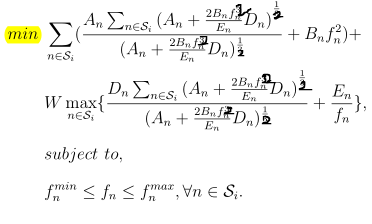Illegal operation: {convex} .^ {0.5}

I try to use CVX to solve a convex problem, this is the problem fomulation:and this is my code:
function [f,beita,cvx_optval] = resource_allocation(Si_size,A,B,W,D,E,f_min,f_max)
cvx_begin
variable f(Si_size)
expressions T1(Si_size)
expressions beita_t(Si_size)
expressions T2(Si_size)
expressions summ
summ = 0
for i = 1:Si_size
T1(i) = (A(i) + 2*B(i)*f(i)*f(i)*D(i)/E(i))^(1/2);
summ = summ + T1(i);
end
for i = 1:Si_size
beita_t(i) = T1(i)/summ
T1(i) = A(i)*inv_pos(beita_t(i))+ B(i)*f(i)*f(i);
T2(i) = D(i)*inv_pos(beita_t(i)) + E(i)inv_pos(f(i))
end
minimize(sum(T1)+W
max(T2))
subject to
f_min <= f <= f_max
cvx_end
T = (A + 2.B.(f.^2).*D./E).^(1/2)
beita = T./sum(T)
disp(f)
disp(beita)
end

I set the value of the parameters randomly as
Si_size = 3; %length(A/B/D/E)
A = [1,2,3];
B = [2,3,4];
W = 2; %a constant
D = [4,5,2];
E = [2,4,6];
f_min = 2;
f_max = 8;

and the error is:
Illegal operation: {convex} .^ {0.5}
(Consider POW_P, POW_POS, or POW_ABS instead.)
.^ (line 55)
z = pow_cvx( x, y, ‘power’ );
^ (line 9)
z = power( x, y );
resource_allocation (line 32)
T1(i) = (A(i) + 2*B(i)*f(i)*f(i)*D(i)/E(i))^(1/2);

i have tried many methods(maybe wrong methods), but still cannot solve it.
so how to express the problem properly in cvx?
I really appreciate your geneous help.

Presuming everything has the needed sign, perhaps you can do something along the lines of representing something of the general form
sqrt(a+b*f^2)
as
norm([sqrt(a);sqrt(b)*f]) .
I’ll leave all the indexing and other details to you.

Oh thank you so much Mark! The error is resolved now.
But A new error has occurred:
Disciplined convex programming error:
Cannot perform the operation: {convex} ./ {convex}
resource_allocation (line 37)
beita_t(i) = T1(i)/summ
I think there might be some other misrepresentations in this code, I would really appreciate it if you could help me with the whole optimization problem!

That is a convex fractional form. I leave it to you to prove your problem is convex, if it indeed it is. You can read about how to deal with linear fractional forms at https://web.stanford.edu/~boyd/cvxbook/bv_cvxbook.pdf . But your problem does not have linear fractional forms.

Cannot perform the operation norm( {mixed convex/constant},2 )

sqrt(a+b*f^2) as
norm([sqrt(a);sqrt(b)*f]) . a is constant ; f is convex

This appears to have been addressed at {convex} .^ {0.5} .